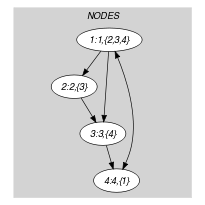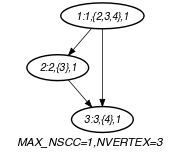## 5.102. cutset

Origin
Constraint

$\mathrm{𝚌𝚞𝚝𝚜𝚎𝚝}\left(\mathrm{𝚂𝙸𝚉𝙴}_\mathrm{𝙲𝚄𝚃𝚂𝙴𝚃},\mathrm{𝙽𝙾𝙳𝙴𝚂}\right)$

Arguments
 $\mathrm{𝚂𝙸𝚉𝙴}_\mathrm{𝙲𝚄𝚃𝚂𝙴𝚃}$ $\mathrm{𝚍𝚟𝚊𝚛}$ $\mathrm{𝙽𝙾𝙳𝙴𝚂}$ $\mathrm{𝚌𝚘𝚕𝚕𝚎𝚌𝚝𝚒𝚘𝚗}\left(\mathrm{𝚒𝚗𝚍𝚎𝚡}-\mathrm{𝚒𝚗𝚝},\mathrm{𝚜𝚞𝚌𝚌}-\mathrm{𝚜𝚒𝚗𝚝},\mathrm{𝚋𝚘𝚘𝚕}-\mathrm{𝚍𝚟𝚊𝚛}\right)$
Restrictions
 $\mathrm{𝚂𝙸𝚉𝙴}_\mathrm{𝙲𝚄𝚃𝚂𝙴𝚃}\ge 0$ $\mathrm{𝚂𝙸𝚉𝙴}_\mathrm{𝙲𝚄𝚃𝚂𝙴𝚃}\le |\mathrm{𝙽𝙾𝙳𝙴𝚂}|$ $\mathrm{𝚛𝚎𝚚𝚞𝚒𝚛𝚎𝚍}$$\left(\mathrm{𝙽𝙾𝙳𝙴𝚂},\left[\mathrm{𝚒𝚗𝚍𝚎𝚡},\mathrm{𝚜𝚞𝚌𝚌},\mathrm{𝚋𝚘𝚘𝚕}\right]\right)$ $\mathrm{𝙽𝙾𝙳𝙴𝚂}.\mathrm{𝚒𝚗𝚍𝚎𝚡}\ge 1$ $\mathrm{𝙽𝙾𝙳𝙴𝚂}.\mathrm{𝚒𝚗𝚍𝚎𝚡}\le |\mathrm{𝙽𝙾𝙳𝙴𝚂}|$ $\mathrm{𝚍𝚒𝚜𝚝𝚒𝚗𝚌𝚝}$$\left(\mathrm{𝙽𝙾𝙳𝙴𝚂},\mathrm{𝚒𝚗𝚍𝚎𝚡}\right)$ $\mathrm{𝙽𝙾𝙳𝙴𝚂}.\mathrm{𝚋𝚘𝚘𝚕}\ge 0$ $\mathrm{𝙽𝙾𝙳𝙴𝚂}.\mathrm{𝚋𝚘𝚘𝚕}\le 1$
Purpose

Consider a digraph $G$ with $n$ vertices described by the $\mathrm{𝙽𝙾𝙳𝙴𝚂}$ collection. Enforces that the subset of kept vertices of cardinality $n-\mathrm{𝚂𝙸𝚉𝙴}_\mathrm{𝙲𝚄𝚃𝚂𝙴𝚃}$ and their corresponding arcs form a graph without circuit.

Example
$\left(\begin{array}{c}1,〈\begin{array}{ccc}\mathrm{𝚒𝚗𝚍𝚎𝚡}-1\hfill & \mathrm{𝚜𝚞𝚌𝚌}-\left\{2,3,4\right\}\hfill & \mathrm{𝚋𝚘𝚘𝚕}-1,\hfill \\ \mathrm{𝚒𝚗𝚍𝚎𝚡}-2\hfill & \mathrm{𝚜𝚞𝚌𝚌}-\left\{3\right\}\hfill & \mathrm{𝚋𝚘𝚘𝚕}-1,\hfill \\ \mathrm{𝚒𝚗𝚍𝚎𝚡}-3\hfill & \mathrm{𝚜𝚞𝚌𝚌}-\left\{4\right\}\hfill & \mathrm{𝚋𝚘𝚘𝚕}-1,\hfill \\ \mathrm{𝚒𝚗𝚍𝚎𝚡}-4\hfill & \mathrm{𝚜𝚞𝚌𝚌}-\left\{1\right\}\hfill & \mathrm{𝚋𝚘𝚘𝚕}-0\hfill \end{array}〉\hfill \end{array}\right)$

The $\mathrm{𝚌𝚞𝚝𝚜𝚎𝚝}$ constraint holds since the vertices of the $\mathrm{𝙽𝙾𝙳𝙴𝚂}$ collection for which the $\mathrm{𝚋𝚘𝚘𝚕}$ attribute is set to 1 correspond to a graph without circuit and since exactly one ($\mathrm{𝚂𝙸𝚉𝙴}_\mathrm{𝙲𝚄𝚃𝚂𝙴𝚃}=1$) vertex has its $\mathrm{𝚋𝚘𝚘𝚕}$ attribute set to 0.

Typical
 $\mathrm{𝚂𝙸𝚉𝙴}_\mathrm{𝙲𝚄𝚃𝚂𝙴𝚃}>0$ $\mathrm{𝚂𝙸𝚉𝙴}_\mathrm{𝙲𝚄𝚃𝚂𝙴𝚃}\le |\mathrm{𝙽𝙾𝙳𝙴𝚂}|$ $|\mathrm{𝙽𝙾𝙳𝙴𝚂}|>1$
Symmetry

Items of $\mathrm{𝙽𝙾𝙳𝙴𝚂}$ are permutable.

Usage

The article [FagesLal03] introducing the $\mathrm{𝚌𝚞𝚝𝚜𝚎𝚝}$ constraint mentions applications from various areas such that deadlock breaking or program verification.

Remark

The undirected version of the $\mathrm{𝚌𝚞𝚝𝚜𝚎𝚝}$ constraint corresponds to the minimum feedback vertex set problem.

Algorithm

The filtering algorithm presented in [FagesLal03] uses graph reduction techniques inspired from Levy and Low [LevyLow88] as well as from Lloyd, Soffa and Wang [LloydSoffaWang88].

Keywords
Arc input(s)

$\mathrm{𝙽𝙾𝙳𝙴𝚂}$

Arc generator
$\mathrm{𝐶𝐿𝐼𝑄𝑈𝐸}$$↦\mathrm{𝚌𝚘𝚕𝚕𝚎𝚌𝚝𝚒𝚘𝚗}\left(\mathrm{𝚗𝚘𝚍𝚎𝚜}\mathtt{1},\mathrm{𝚗𝚘𝚍𝚎𝚜}\mathtt{2}\right)$

Arc arity
Arc constraint(s)
 $•$$\mathrm{𝚒𝚗}_\mathrm{𝚜𝚎𝚝}$$\left(\mathrm{𝚗𝚘𝚍𝚎𝚜}\mathtt{2}.\mathrm{𝚒𝚗𝚍𝚎𝚡},\mathrm{𝚗𝚘𝚍𝚎𝚜}\mathtt{1}.\mathrm{𝚜𝚞𝚌𝚌}\right)$ $•\mathrm{𝚗𝚘𝚍𝚎𝚜}\mathtt{1}.\mathrm{𝚋𝚘𝚘𝚕}=1$ $•\mathrm{𝚗𝚘𝚍𝚎𝚜}\mathtt{2}.\mathrm{𝚋𝚘𝚘𝚕}=1$
Graph property(ies)
 $•$$\mathrm{𝐌𝐀𝐗}_\mathrm{𝐍𝐒𝐂𝐂}$$\le 1$ $•$$\mathrm{𝐍𝐕𝐄𝐑𝐓𝐄𝐗}$$=|\mathrm{𝙽𝙾𝙳𝙴𝚂}|-\mathrm{𝚂𝙸𝚉𝙴}_\mathrm{𝙲𝚄𝚃𝚂𝙴𝚃}$

Graph class
 $•$$\mathrm{𝙰𝙲𝚈𝙲𝙻𝙸𝙲}$ $•$$\mathrm{𝙽𝙾}_\mathrm{𝙻𝙾𝙾𝙿}$

Graph model

We use a set of integers for representing the successors of each vertex. Because of the arc constraint, all arcs such that the $\mathrm{𝚋𝚘𝚘𝚕}$ attribute of one extremity is equal to 0 are eliminated; Therefore all vertices for which the $\mathrm{𝚋𝚘𝚘𝚕}$ attribute is equal to 0 are also eliminated (since they will correspond to isolated vertices). The graph property $\mathrm{𝐌𝐀𝐗}_\mathrm{𝐍𝐒𝐂𝐂}$ $\le$ 1 enforces the size of the largest strongly connected component to not exceed 1; Therefore, the final graph cannot contain any circuit.

Part (A) of Figure 5.102.1 shows the initial graph from which we have chosen to start. It is derived from the set associated with each vertex. Each set describes the potential values of the $\mathrm{𝚜𝚞𝚌𝚌}$ attribute of a given vertex. Part (B) of Figure 5.102.1 gives the final graph associated with the Example slot. Since we use the $\mathrm{𝐍𝐕𝐄𝐑𝐓𝐄𝐗}$ graph property, the vertices of the final graph are stressed in bold. The $\mathrm{𝚌𝚞𝚝𝚜𝚎𝚝}$ constraint holds since the final graph does not contain any circuit and since the number of removed vertices $\mathrm{𝚂𝙸𝚉𝙴}_\mathrm{𝙲𝚄𝚃𝚂𝙴𝚃}$ is equal to 1.

##### Figure 5.102.1. Initial and final graph of the $\mathrm{𝚌𝚞𝚝𝚜𝚎𝚝}$ set constraint(a) (b)